# Olympiad Test: Completion Of Incomplete Pattern - 1

## 10 Questions MCQ Test Mathematical Olympiad Class 8 | Olympiad Test: Completion Of Incomplete Pattern - 1

Description
Attempt Olympiad Test: Completion Of Incomplete Pattern - 1 | 10 questions in 20 minutes | Mock test for Class 8 preparation | Free important questions MCQ to study Mathematical Olympiad Class 8 for Class 8 Exam | Download free PDF with solutions
QUESTION: 1

Solution:
QUESTION: 2

Solution:
QUESTION: 3

### Direction: In the following problems, select a figure from amongst the four options which when placed in the blank space of figure (X) would complete the pattern.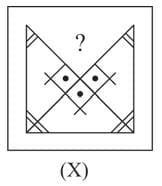Solution:
QUESTION: 4

Direction: In the following problems, select a figure from amongst the four options which when placed in the blank space of figure (X) would complete the pattern.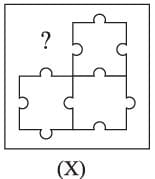Solution:
QUESTION: 5

Direction: In the following problems, select a figure from amongst the four options which when placed in the blank space of figure (X) would complete the pattern.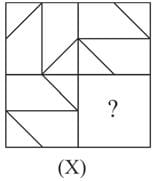Solution:
QUESTION: 6

Direction: In the following problems, select a figure from amongst the four options which when placed in the blank space of figure (X) would complete the pattern.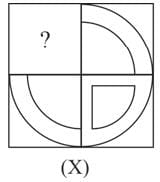Solution:
QUESTION: 7

Direction: In the following problems, select a figure from amongst the four options which when placed in the blank space of figure (X) would complete the pattern.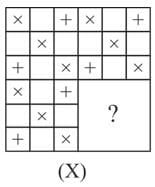Solution:
QUESTION: 8

Direction: In the following problems, select a figure from amongst the four options which when placed in the blank space of figure (X) would complete the pattern.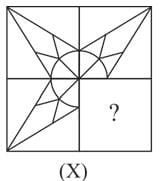Solution:
QUESTION: 9

Direction: In the following problems, select a figure from amongst the four options which when placed in the blank space of figure (X) would complete the pattern.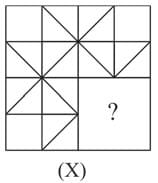Solution:
QUESTION: 10

Direction: In the following problems, select a figure from amongst the four options which when placed in the blank space of figure (X) would complete the pattern.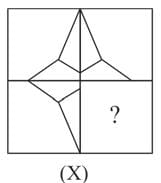Solution:Use Code STAYHOME200 and get INR 200 additional OFF Use Coupon Code